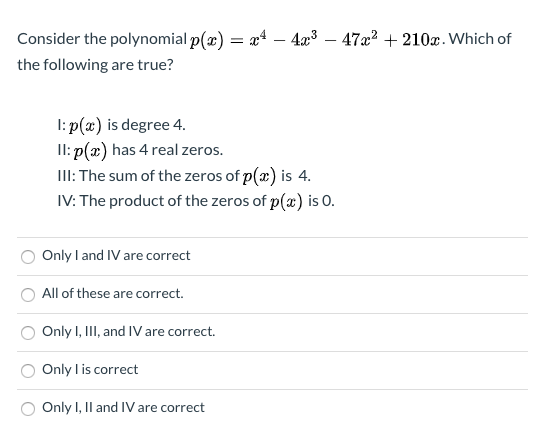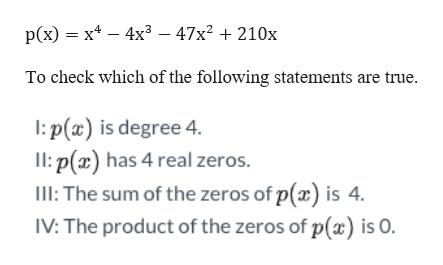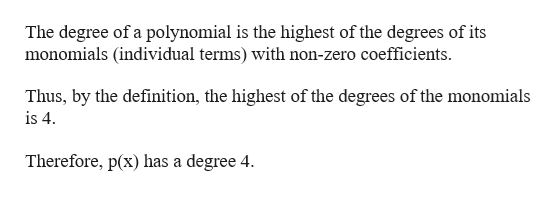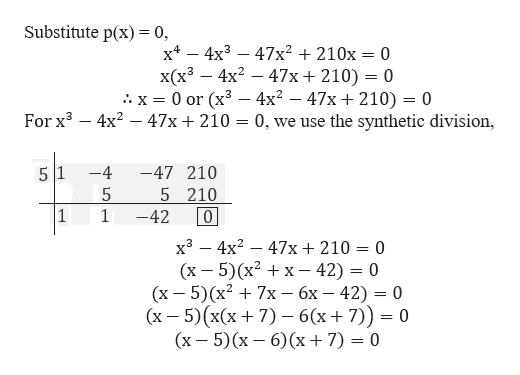# Consider the polynomial p(x) = x* – 4æ3- 47x2 + 210x. Which ofthe following are true?I: p(x) is degree 4.Il: p(x) has 4 real zeros.III: The sum of the zeros of p(2) is 4.IV: The product of the zeros of p(x) is O.Only I and IV are correctAll of these are correct.Only I, III, and IV are correct.Only l is correctOnly I, Il and IV are correct

Question
32 viewshelp_outlineImage TranscriptioncloseConsider the polynomial p(x) = x* – 4æ3 - 47x2 + 210x. Which of the following are true? I: p(x) is degree 4. Il: p(x) has 4 real zeros. III: The sum of the zeros of p(2) is 4. IV: The product of the zeros of p(x) is O. Only I and IV are correct All of these are correct. Only I, III, and IV are correct. Only l is correct Only I, Il and IV are correct fullscreen
check_circle

Step 1

Given a polynomialhelp_outlineImage Transcriptionclosep(x) = x* – 4x³ – 47x² + 210x To check which of the following statements are true. I: p(x) is degree 4. Il: p(x) has 4 real zeros. III: The sum of the zeros of p(x) is 4. IV: The product of the zeros of p(x) is 0. fullscreen
Step 2

Note:help_outlineImage TranscriptioncloseThe degree of a polynomial is the highest of the degrees of its monomials (individual terms) with non-zero coefficients. Thus, by the definition, the highest of the degrees of the monomials is 4. Therefore, p(x) has a degree 4. fullscreen
Step 3

Next, we find the zeros of t...help_outlineImage TranscriptioncloseSubstitute p(x) = 0, х* - 4x3 – 47x? + 210x = 0 x(x³ – 4x? – 47x+ 210) = 0 .: x = 0 or (x³ –- 4x? – 47x + 210) = 0 4x? – 47x + 210 = 0, we use the synthetic division, %3D For x3 -4 -47 210 5 210 -42 x3 – 4x? – 47x+210 = 0 (х — 5)(x2 + x — 42) — 0 (х — 5)(х2 + 7х — бх — 42) 3 0 (x – 5)(x(x+ 7) – 6(x+7)) = 0 (х — 5) (х — 6) (х + 7) %3D 0 fullscreen

### Want to see the full answer?

See Solution

#### Want to see this answer and more?

Solutions are written by subject experts who are available 24/7. Questions are typically answered within 1 hour.*

See Solution
*Response times may vary by subject and question.
Tagged in

### Other